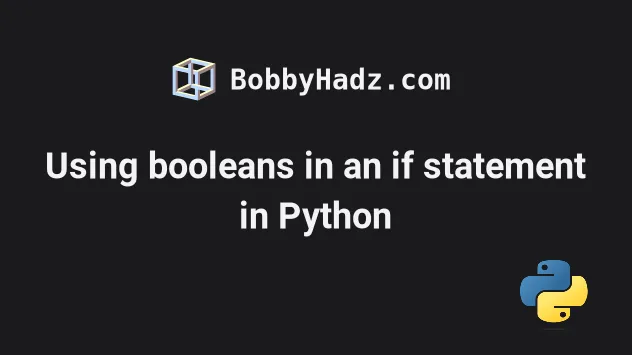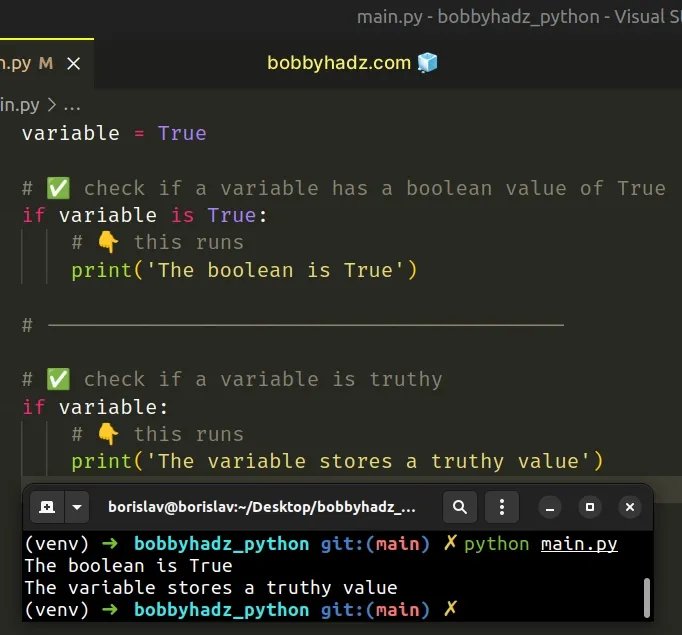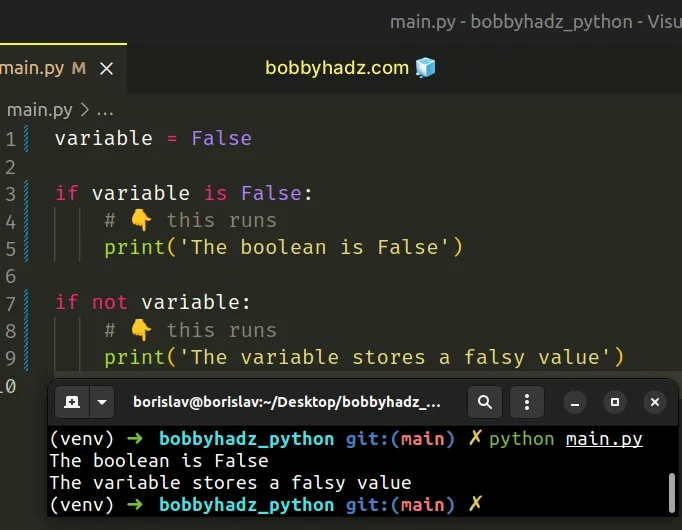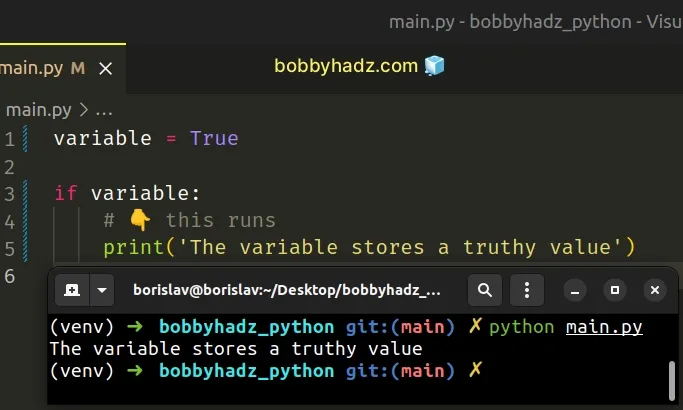# Using booleans in an if statement in PythonLast updated: Feb 20, 2023
3 min## #Using booleans in an if statement in Python

Use the `is` operator to check for a boolean value in an if statement, e.g. `if variable is True:`.

The `is` operator will return `True` if the condition is met and `False` otherwise.

main.py
```Copied!```variable = True

# ✅ check if a variable has a boolean value of True
if variable is True:
# 👇️ this runs
print('The boolean is True')

# ------------------------------------------

# ✅ check if a variable is truthy
if variable:
# 👇️ this runs
print('The variable stores a truthy value')
``````The first example checks if the variable stores a `True` boolean value.

You should use the `is` operator when you need to check if a variable stores a boolean value or `None`.

Use the equality operators (equals `==` and not equals `!=`) when you need to check if a value is equal to another value, e.g. `'abc' == 'abc'`.

In short, use the `is` operator with built-in constants like `True`, `False` and `None`.

## #Checking for False or a Falsy value

You can use the same approach when checking for `False` or a falsy value in an `if` statement.

main.py
```Copied!```variable = False

if variable is False:
# 👇️ this runs
print('The boolean is False')

if not variable:
# 👇️ this runs
print('The variable stores a falsy value')
``````Note that checking for the boolean values `True` and `False` is very different than checking for a truthy or falsy value.

## #Checking for a Truthy Value in an if statement

Here is how we would check for a truthy value in an `if` statement.

main.py
```Copied!```variable = True

if variable:
# 👇️ this runs
print('The variable stores a truthy value')
``````The falsy values in Python are:

• constants defined to be falsy: `None` and `False`.
• `0` (zero) of any numeric type
• empty sequences and collections: `""` (empty string), `()` (empty tuple), `[]` (empty list), `{}` (empty dictionary), `set()` (empty set), `range(0)` (empty range).
All other values are truthy, so the `if` block runs if any other value is stored in the variable.

Here are some examples.

main.py
```Copied!```if 'hello':
print('this runs ✅')

if ['a', 'b']:
print('This runs ✅')

if '':
print('this does NOT run ⛔️')

if 0:
print('this does NOT run ⛔️')
``````

`if not X` returns the negation of `if X`. So with `if not X`, we check if `X` stores a falsy value.

You will often have to use multiple conditions in a single `if` statement.

You can do that by using the boolean AND or boolean OR operators.

main.py
```Copied!```if True and True:
print('This runs ✅')

if True and False:
print('This does NOT run ⛔️')

# --------------------------------------

if True or False:
print('This runs ✅')

if False or False:
print('This does NOT run ⛔️')
``````

The first two examples use the boolean AND operator and the second two examples use the boolean `or` operator.

When using the boolean `and` operator, the expressions on the left and right-hand sides have to be truthy for the `if` block to run.

In other words, both conditions have to be met for the `if` block to run.

main.py
```Copied!```if True and True:
print('This runs ✅')

if True and False:
print('This does NOT run ⛔️')
``````

When using the boolean `or` operator, either of the conditions has to be met for the `if` block to run.

main.py
```Copied!```if True or False:
print('This runs ✅')

if False or False:
print('This does NOT run ⛔️')
``````

If the expression on the left or right-hand side evaluates to a truthy value, the `if` block runs.

I've also written an article on how to check for multiple conditions in an if statement.